# Quiz 14: Simulation

Calculate the benefit cost ratio of the project, as shown below: Step1: Estimate the total of benefits accruing from the projects as shown under: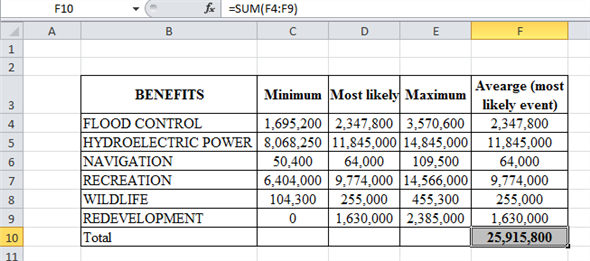The average or the most likely event is taken as the mean of the benefit for each criterion. Sum of the benefits is the total of the benefit from each 6 criteria's. Step2: Estimate the total costs in undertaking the project, as shown below: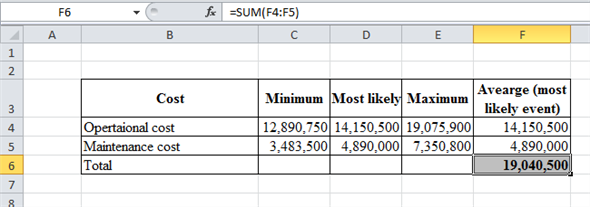The total cost is the sum of the cost accruing from each operational and maintenance source. Step3: Calculate the Benefit cost ratio by using the formula, as shown below: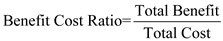B/C ratio = 25,915,800/19,040,500 B/C ratio = 1.361 Step 5: Estimate the probability of the Benefit cost ratio greater than 1, is shown as below: P (x = 1)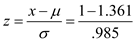=-2.48 Calculate the probability for the given z value table. The probability for z 2.48 is 0.4934. Thus, the probability of benefit cost ratio greater than 1 = 0.493+0.5 = 0.993
To determine the loss of revenue due to machine break down, using simulation is shown as follows: Step 1: Develop the probability distribution for machine repair time, as shown below: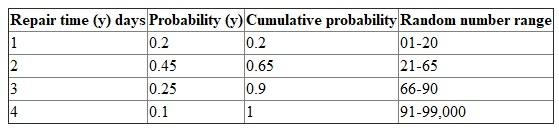Continuous probability function for time between breakdowns is given as: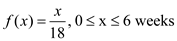Here, x = time between machine breakdown The equation for generating x, given random number r for the probability distribution is: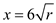…… (1) Here, x = time between machine breakdown r= random numbers Also, the z (loss of revenue from the repair) is given by: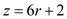…… (2) Here, z= function of loss r= random number Step 2: Generate the random numbers for the break down and repair time as shown below: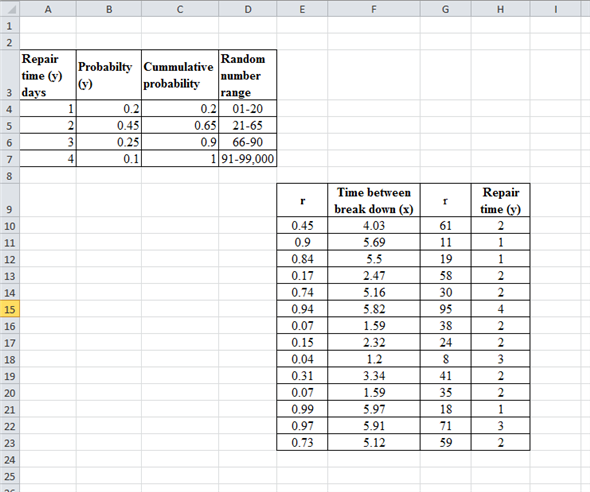Generate the random numbers by entering the formula in the cell as =RAND ( ) and calculate for both breakdown and repair time. x (breakdown time) is calculated using the formula in equation (2), which is as follows: x = 6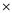vr =6v0.45 =4.03 Similarly, calculate the values for all the random variable. Calculate y (repair time) from the cumulative probability, i.e. Random number 61 lies in the range of 21-65 which has the repair time as 2. Step3: Obtain the random numbers for the loss accruing from the breakdown, as shown below: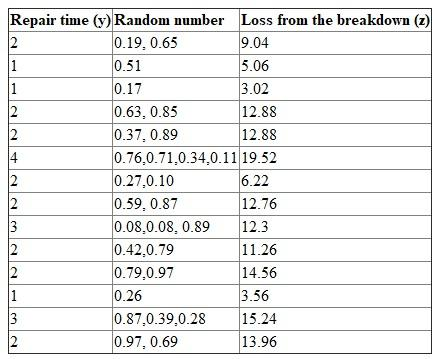As per the repair time in days, the random numbers are generated for each day. Such that for 2 days of repair time there are 2 random numbers associated with it. For repair time as 2, calculate the value of z using equation (2) as follows: z = 6r+2 =60.19 +2 = 3.14 z = 60.65+2 =5.90 Thus, the cumulative of the loss from the breakdown is 9.04 (3.14+5.09). Similarly the loss for each random number is calculated. Step4: Derive the total loss of revenue due to the machine break down, as shown below: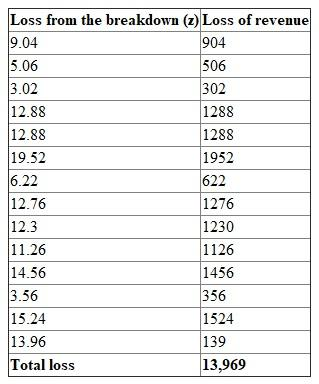Thus, since the loss from the revenue is greater than 12,000 they should purchase a backup copier machine.